# Select one color with matplotlib

This post aims to describe how to set a color with the matplotlib library of python. These techniques also work for most of the python libraries, since most of them are build on top of matplotlib. Note that the next post is dedicated to the choice and utilisation of color palettes.

## Name

The most common way to call a color is by its name. It is highly advised to avoid the basic ‘blue’, ‘red’, ‘green’, etc. since they seem quite ugly in charts. There are so many other nice possibilities. See the figure which shows all the possibilities in this page.(Thanks to the Matplotlib documentation for their work).

``````# libraries
from matplotlib import pyplot as plt
import pandas as pd
import numpy as np

# dataset
df=pd.DataFrame({'x_pos': range(1,101), 'y_pos': np.random.randn(100)*15+range(1,101)})

# plot
plt.plot( 'x_pos', 'y_pos', data=df, marker='o', color='mediumvioletred')

# show the graph
plt.show()``````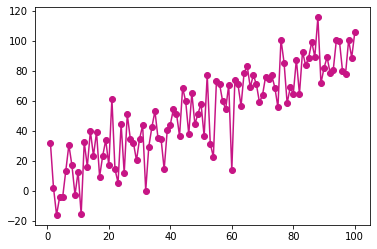## Abbreviation

It is also possible to call a color with the abbreviation of its name:

``````# libraries
from matplotlib import pyplot as plt
import pandas as pd
import numpy as np

# dataset
df=pd.DataFrame({'x_pos': range(1,101), 'y_pos': np.random.randn(100)*15+range(1,101)})

# Plot
plt.plot( 'x_pos', 'y_pos', data=df, marker='o', color='c')

# Show the graph
plt.show()``````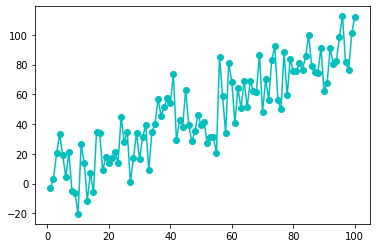## Hex Code

This example shows how to use hex codes to call a name:

``````# library
from matplotlib import pyplot as plt
import pandas as pd
import numpy as np

# dataset
df=pd.DataFrame({'x_pos': range(1,101), 'y_pos': np.random.randn(100)*15+range(1,101)})

# plot
plt.plot( 'x_pos', 'y_pos', data=df, marker='o', color='#8f9805')

# show the graph
plt.show()``````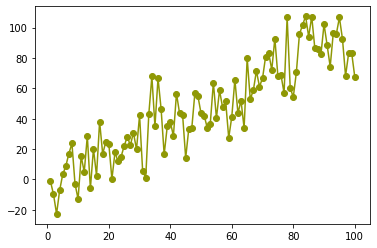## RGB

Here is an example which passes an RGB colors to the plot:

``````# libraries
from matplotlib import pyplot as plt
import pandas as pd
import numpy as np

# dataset
df=pd.DataFrame({'x_pos': range(1,101), 'y_pos': np.random.randn(100)*15+range(1,101)})

# plot
plt.plot( 'x_pos', 'y_pos', data=df, marker='o', color=(0.9, 0.2, 0.5, 0.2))

# show the graph
plt.show()``````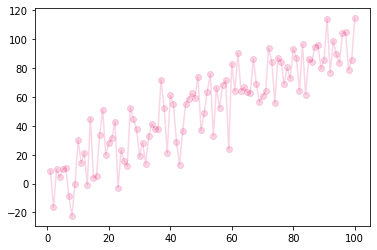## Black and White

Here is an example of black and white plot:

``````# libraries
from matplotlib import pyplot as plt
import pandas as pd
import numpy as np

# dataset
df=pd.DataFrame({'x_pos': range(1,101), 'y_pos': np.random.randn(100)*15+range(1,101)})

# plot
plt.plot( 'x_pos', 'y_pos', data=df, marker='o', color='0.9')

# show the graph
plt.show()``````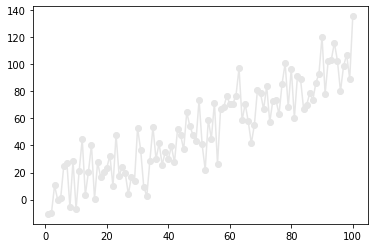## Transparency

You can change the color transparency with the `alpha` parameter:

``````# libraries
from matplotlib import pyplot as plt
import pandas as pd
import numpy as np

# dataset
df=pd.DataFrame({'x_pos': range(1,101), 'y_pos': np.random.randn(100)*15+range(1,101)})

# plot
plt.plot( 'x_pos', 'y_pos', data=df, marker='o', color='blue', alpha=0.3)

# show the graph
plt.show()``````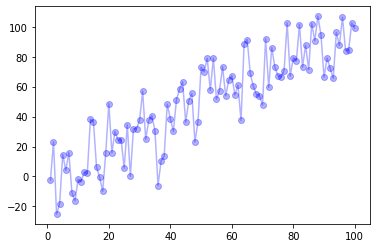## Contact & Edit

👋 This document is a work by Yan Holtz. Any feedback is highly encouraged. You can fill an issue on Github, drop me a message onTwitter, or send an email pasting `yan.holtz.data` with `gmail.com`.